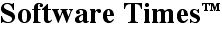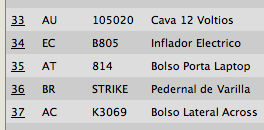Downloads...

January 7, 2012

# JavaScript: Zebra Striping CSS TablesA lot has been written about zebra striping HTML tables when the table is built using the <table...> tag but I could not find any references to zebra striping tables built with CSS tables:
``````
/*******  css tables  *******/

.table {
display:table;
}
.row {
display:table-row;
}
.cell {
display:table-cell;
}
.backgroundLight {
background-color:#ccc;
}
.backgroundDark {
background-color:#ddd;
}
``````
and I had to build my own. The above styles are at the core of the striping, the JavaScript will rely on these classes to do its work. A CSS table looks like this:
``````
<div id="table" class="table">
<div class="row backgroundLight">
<div class="cell">
<p>First row, first cell stuff</p>
</div>
<div class="cell">
<p>First row, second cell stuff</p>
</div>
</div>
<div class="row backgroundDark">
<div class="cell">
<p>Second row, first cell stuff</p>
</div>
<div class="cell">
<p>Second row, second cell stuff</p>
</div>
</div>
</div>
``````
I could use the table's id to retrieve it from the DOM node list
``````
list = document.getElementById('table');
``````
but that would get all the nodes in the table and all I need are the divs, so I add getElementsByTagName('div')
``````
list = document.getElementById('table').getElementsByTagName('div');
``````
Now we have all the divs but we will work only with the ones class row. For that we need to loop through the retrieved div node list to find the ones whose class attribute starts with 'row'
``````
// loop through css table
count = list.length;
for(i = 0; i < count; i++) {

// get ith element from the list
elem = list(i);

// get the element's class attribute (can't use 'class' a reserved word)
clas = elem.getAttribute('class');

// if element's class is row, process, else ignore
if(clas.slice(0,3) == 'row') {
// here is where we do the magic, explained below
}
}
``````
Now that we have the row divs, we want to set their class alternatively to 'row backgroundLight' and 'row backgroundDark' To do so, I'm going to use a toggled even/odd flag
``````
if(clas.slice(0,3) == 'row') {

// if even, light, else, dark
elem.setAttribute("class", even ? light : dark);

// toggle even/odd (true/false)
even = ! even;
}
``````
That's it! Here is the entire code. Since I want to reuse it for several tables it's written as a function:
``````
// zebra stripe rows of CSS table with id='table'
zebraStripe('table');

--------------------------

function zebraStripe(table) {

// set variables
var list, light, dark, even, count, elem, clas;
light = 'row backgroundLight';
dark  = 'row backgroundDark';
even = true;

// get table's div nodes
list = document.getElementById(table).getElementsByTagName('div');

// loop through css table
count = list.length;
for(i = 0; i < count; i++) {

// get ith element from the node list
elem = list(i);

// get the element's class attribute (can't use 'class' a reserved word)
clas = elem.getAttribute('class');

// if element's class is row, process, else ignore
if(clas.slice(0,3) == 'row') {

// if even, light, else, dark
elem.setAttribute("class", even ? light : dark);

// toggle even/odd (true/false)
even = ! even;
}
}
}
``````

Enjoy!
Denny Schlesinger

Test drive zebraStripe (in a new window)
Download zebraStripe (1.4 KB)

Share this article with your followersCopyright © Software Times, 2000 - 2013. All rights reserved.
Last updated February 12, 2013.